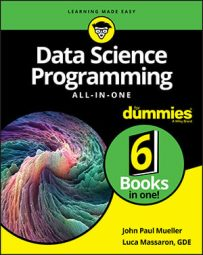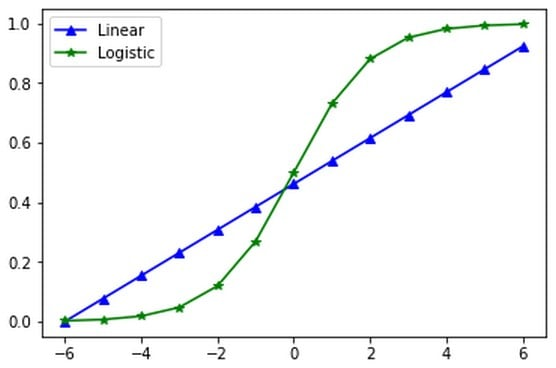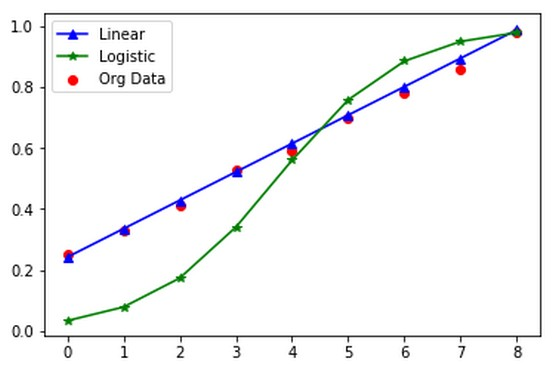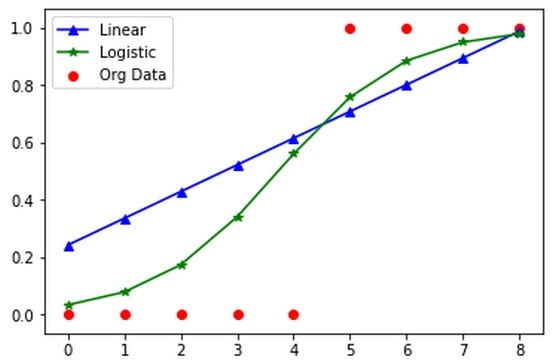##### Data Science Programming All-in-One For DummiesBoth linear and logistic regression see a lot of use in data science but are commonly used for different kinds of problems. You need to know and understand both types of regression to perform a full range of data science tasks.

Of the two, logistic regression is harder to understand in many respects because it necessarily uses a more complex equation model. The following information gives you a basic overview of how linear and logistic regression differ.

## The equation model

Any discussion of the difference between linear and logistic regression must start with the underlying equation model. The equation for linear regression is straightforward.
`y = a + bx`
You may see this equation in other forms and you may see it called ordinary least squares regression, but the essential concept is always the same. Depending on the source you use, some of the equations used to express logistic regression can become downright terrifying unless you’re a math major. However, the start of this discussion can use one of the simplest views of logistic regression:
`p = f(a + bx)`
`>p`, is equal to the logistic function, f, applied to two model parameters, `a` and `b`, and one explanatory variable, `x`. When you look at this particular model, you see that it really isn’t all that different from the linear regression model, except that you now feed the result of the linear regression through the logistic function to obtain the required curve.

The output (dependent variable) is a probability ranging from 0 (not going to happen) to 1 (definitely will happen), or a categorization that says something is either part of the category or not part of the category. (You can also perform multiclass categorization, but focus on the binary response for now.) The best way to view the difference between linear regression output and logistic regression output is to say that the following:

• Linear regression is continuous. A continuous value can take any value within a specified interval (range) of values. For example, no matter how closely the height of two individuals matches, you can always find someone whose height fits between those two individuals. Examples of continuous values include:
• Height
• Weight
• Waist size
• Logistic regression is discrete. A discrete value has specific values that it can assume. For example, a hospital can admit only a specific number of patients in a given day. You can’t admit half a patient (at least, not alive). Examples of discrete values include:
• Number of people at the fair
• Number of jellybeans in the jar
• Colors of automobiles produced by a vendor

## The logistic function

Of course, now you need to know about the logistic function. You can find a variety of forms of this function as well, but here’s the easiest one to understand:
`f(x) = e<sup>x</sup> / e<sup>x</sup> + 1`
You already know about `f`, which is the logistic function, and `x` equals the algorithm you want to use, which is `a + bx `in this case. That leaves `e`, which is the natural logarithm and has an irrational value of 2.718, for the sake of discussion (check out a better approximation of the whole value). Another way you see this function expressed is
`f(x) = 1 / (1 + e<sup>-x</sup>)`
Both forms are correct, but the first form is easier to use. Consider a simple problem in which `a`, the y-intercept, is 0, and `">b`, the slope, is 1. The example uses `x` values from –6 to 6. Consequently, the first `f(x)` value would look like this when calculated (all values are rounded):
```
(1) e<sup>-6</sup> / (1 + e<sup>-6</sup>)
(2) 0.00248 / 1 + 0.00248
(3) 0.002474```
As you might expect, an `x`value of 0 would result in an `f(x)` value of 0.5, and an `x` value of 6 would result in an `f(x)` value of 0.9975. Obviously, a linear regression would show different results for precisely the same `x` values. If you calculate and plot all the results from both logistic and linear regression using the following code, you receive a plot like the one below.
```import matplotlib.pyplot as plt
%matplotlib inline
from math import exp

x_values = range(-6, 7)
lin_values = [(0 + 1*x) / 13 for x in range(0, 13)]
log_values = [exp(0 + 1*x) / (1 + exp(0 + 1*x))
for x in x_values]

plt.plot(x_values, lin_values, 'b-^')
plt.plot(x_values, log_values, 'g-*')
plt.legend(['Linear', 'Logistic'])
plt.show()```Contrasting linear to logistic regression.

This example relies on list comprehension to calculate the values because it makes the calculations clearer. The linear regression uses a different numeric range because you must normalize the values to appear in the 0 to 1 range for comparison. This is also why you divide the calculated values by 13. The `exp(x)` call used for the logistic regression raises `e` to the power of `x`, `e<sup>x</sup>`, as needed for the logistic function.

The model discussed here is simplified, and some math majors out there are probably throwing a temper tantrum of the most profound proportions right now. The Python or R package you use will actually take care of the math in the background, so really, what you need to know is how the math works at a basic level so that you can understand how to use the packages. This section provides what you need to use the packages. However, if you insist on carrying out the calculations the old way, chalk to chalkboard, you’ll likely need a lot more information.

## The problems that logistic regression solves

You can separate logistic regression into several categories. The first is simple logistic regression, in which you have one dependent variable and one independent variable, much as you see in simple linear regression. However, because of how you calculate the logistic regression, you can expect only two kinds of output:
• Classification: Decides between two available outcomes, such as male or female, yes or no, or high or low. The outcome is dependent on which side of the line a particular data point falls.
• Probability: Determines the probability that something is true or false. The values true and false can have specific meanings. For example, you might want to know the probability that a particular apple will be yellow or red based on the presence of yellow and red apples in a bin.

## Fit the curve

As part of understanding the difference between linear and logistic regression, consider this grade prediction problem, which lends itself well to linear regression. In the following code, you see the effect of trying to use logistic regression with that data:
```x1 = range(0,9)
y1 = (0.25, 0.33, 0.41, 0.53, 0.59,
0.70, 0.78, 0.86, 0.98)
plt.scatter(x1, y1, c='r')

lin_values = [0.242 + 0.0933*x for x in x1]
log_values = [exp(0.242 + .9033*x) /
(1 + exp(0.242 + .9033*x))
for x in range(-4, 5)]

plt.plot(x1, lin_values, 'b-^')
plt.plot(x1, log_values, 'g-*')
plt.legend(['Linear', 'Logistic', 'Org Data'])
plt.show()```
The example has undergone a few changes to make it easier to see precisely what is happening. It relies on the same data that was converted from questions answered correctly on the exam to a percentage. If you have 100 questions and you answer 25 of them correctly, you have answered 25 percent (0.25) of them correctly. The values are normalized to produce values between 0 and 1 percent.Considering the approach to fitting the data.

As you can see from the image above, the linear regression follows the data points closely. The logistic regression doesn’t. However, logistic regression often is the correct choice when the data points naturally follow the logistic curve, which happens far more often than you might think. You must use the technique that fits your data best, which means using linear regression in this case.

## A pass/fail example

An essential point to remember is that logistic regression works best for probability and classification. Consider that points on an exam ultimately predict passing or failing the course. If you get a certain percentage of the answers correct, you pass, but you fail otherwise. The following code considers the same data used for the example above, but converts it to a pass/fail list. When a student gets at least 70 percent of the questions correct, success is assured.
```y2 = [0 if x < 0.70 else 1 for x in y1]
plt.scatter(x1, y2, c='r')

lin_values = [0.242 + 0.0933*x for x in x1]
log_values = [exp(0.242 + .9033*x) /
(1 + exp(0.242 + .9033*x))
for x in range(-4, 5)]

plt.plot(x1, lin_values, 'b-^')
plt.plot(x1, log_values, 'g-*')
plt.legend(['Linear', 'Logistic', 'Org Data'])
plt.show()```
This is an example of how you can use list comprehensions in Python to obtain a required dataset or data transformation. The list comprehension for `y2` starts with the continuous data in `y1` and turns it into discrete data. Note that the example uses precisely the same equations as before. All that has changed is the manner in which you view the data, as you can see below.Contrasting linear to logistic regression.

Because of the change in the data, linear regression is no longer the option to choose. Instead, you use logistic regression to fit the data. Take into account that this example really hasn’t done any sort of analysis to optimize the results. The logistic regression fits the data even better if you do so.